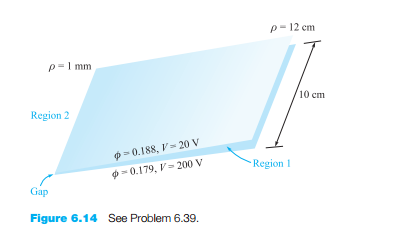### Create an Account

Already have account?

### Forgot Your Password ?

Home / Questions / The conducting planes 2x 3y 12 and 2x 3y 18 are at potentials of 100 V and 0 respectiv...

# The conducting planes 2x 3y 12 and 2x 3y 18 are at potentials of 100 V and 0 respectively Let ϵ ϵ0 and find

The conducting planes 2x + 3y = 12 and 2x + 3y = 18 are at potentials of 100 V and 0, respectively. Let ϵ = ϵ0 and find (a) V at P(5, 2, 6); (b) E at P.

2. The derivation of Laplace’s and Poisson’s equations assumed constant permittivity, but there are cases of spatially varying permittivity in which the equations will still apply. Consider the vector identity, ∇ · (ψG) = G · ∇ψ + ψ∇ · G, where ψ and G are scalar and vector functions, respectively.Determine a general rule on the allowed directions in which ϵ may vary with respect to the local electric field.

Jun 14 2020 View more View Less

#### Answer (Solved)Subscribe To Get Solution